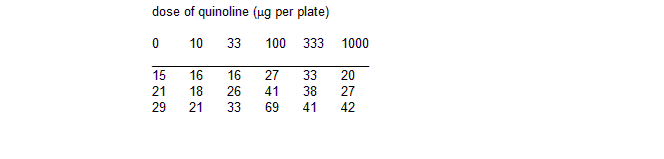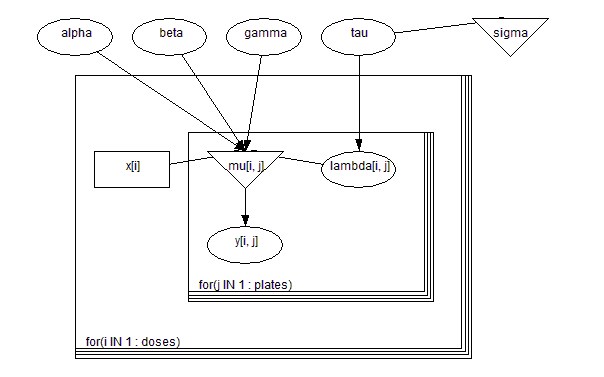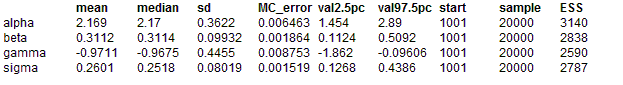#### Salm: extra - Poisson variation in dose - response study

Breslow (1984) analyses some mutagenicity assay data (shown below) on salmonella in which three plates have been processed at each dose i of quinoline and the number of revertant colonies of TA98 Salmonella measured. A certain dose-response curve is suggested by theory.This is assumed to be a random effects Poisson model allowing for over-dispersion. Let xi be the dose on the plates i1, i2 and i3. Then we assume

yij ~ Poisson(μij)

log(μij) = α + β log(xi + 10) + γxi + λij

λij ~ Normal(0, τ)

α , β , γ , τ are given independent ``noninformative'' priors. The appropriate graph is shown

Graphical model for salm exampleBUGS language for salm example

model
{
for( i in 1 : doses ) {
for( j in 1 : plates ) {
y[i , j] ~ dpois(mu[i , j])
log(mu[i , j]) <- alpha + beta * log(x[i] + 10) +
gamma * x[i] / 1000 + lambda[i , j]
lambda[i , j] ~ dnorm(0.0, tau)
}
}
alpha ~ dnorm(0.0,1.0E-6)
beta ~ dnorm(0.0,1.0E-6)
gamma ~ dnorm(0.0,1.0E-6)
tau ~ dgamma(0.001, 0.001)
sigma <- 1 / sqrt(tau)
}

##### Data
``` list(doses = 6, plates = 3,      y = structure(.Data = c(15,21,29,16,18,21,16,26,33,27,41,60,33,38,41,20,27,42),                     .Dim = c(6, 3)),      x = c(0, 10, 33, 100, 333, 1000)) ```
##### Inits for chain 1
``` list(alpha = 0, beta = 0, gamma = 0, tau = 0.1) ```

##### Inits for chain 2
``` list(alpha = 1.0, beta = 1.0, gamma = 0.01, tau = 1.0) ```
ResultsThese estimates can be compared with the quasi-likelihood estimates of Breslow (1984) who reported α = 2.203 +/- 0.363, β = 0.311 +/- 0.099, γ = -9.74E-4 +/- 4.37E-4, σ = 0.268